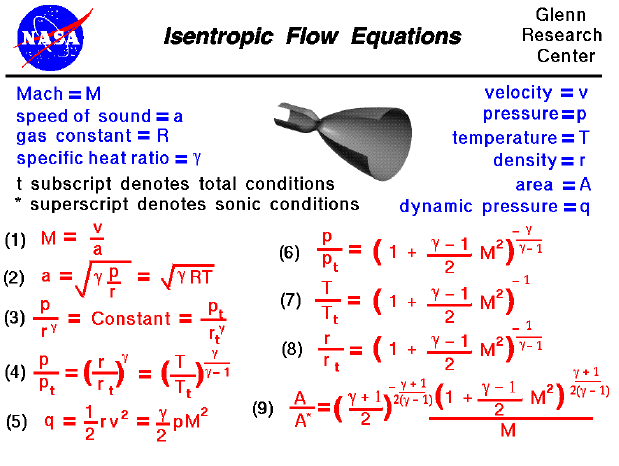A text only version of this slide is available which gives all of the flow equations. An interactive Java applet is also available which solves the various equations shown here.

As a gas is forced through a tube, the gas molecules are deflected by the walls of the tube. If the speed of the gas is much less than the speed of sound of the gas, the density of the gas remains constant. However, if the speed of the flow is nearer the speed of sound we must consider compressibility effects on the gas. The density of the gas will vary locally as the gas is compressed by the walls of the tube. Regardless of the speed, the flow conditions can be determined by conserving mass, momentum, and energy in the flow. For compressible flows with a smooth, gradual flow turning, the change in flow properties are given by the isentropic flow relations which are presented on this slide. Isentropic means constant entropy which implies a reversible process from the second law of thermodynamics.

On this slide we have collected many of the important equations which describe an isentropic flow. The derivation of the equations is given on a separate slide. Beginning at the top left, equation (1), the Mach number is the ratio of the speed of the flow (V) to the speed of sound (a). The speed of sound, in turn, depends on the properties of the gas, as shown in equation (2). As shown on the derivation slide, if we begin with the entropy equations for a gas, it can be shown that the pressure and density of an isentropic flow are related as shown in the first part of equation (3). We can determine the value of the constant by defining total conditions to be the pressure and density when the flow is brought to rest isentropically. The "t" subscript used in many of these equations stands for "total conditions". (You probably already have some idea of total conditions from experience with Bernoulli's equation). Using the equation of state, we can easily derive equations (4) and (5) from equation (3). Equations (6), (7), and (8) are derived by considering the total enthalpy, of the flow. While equation (9) can be derived from the compressible mass flow equation and defining the starred conditions to occur when the flow is choked and the Mach number is equal to one. Notice the important role that the Mach number plays in all the equations on the right side of this slide. If the Mach number of the flow is determined, all of the other flow relations can be determined. Similarly, determining any flow relation (pressure ratio for example) will fix the Mach number and set all the other flow conditions.

The isentropic flow relations apply to gradual changes in the flow and the same equations can be used to describe the flow through a tube or flow past an object. If, however, the turning of the flow is abrupt and decreases the flow area, shock waves are generated and the isentropic relations are no longer valid because the flow is irreversible . The flow is then governed by the oblique or normal shock relations depending on the free stream Mach number and the amount of flow turning. The equations describing oblique and normal shocks and isentropic flows were published in a NACA report (NACA-1135) in 1951.

Guided Tours

Navigation..

Beginner's Guide to Aerodynamics
Beginner's Guide to Propulsion
Beginner's Guide to Model Rockets
Beginner's Guide to Kites
Beginner's Guide to Aeronautics

Go to...

Beginner's Guide Home Page

byTom Benson
Please send suggestions/corrections to: benson@grc.nasa.gov